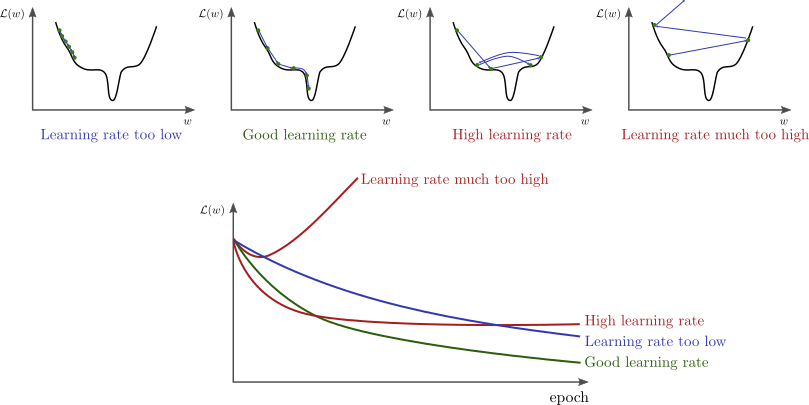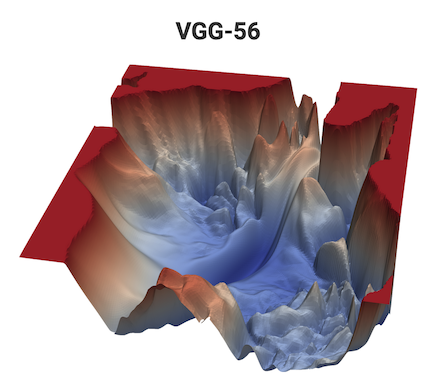One of the first questions you’ll ask yourself when starting to train a neural network is “what do I set the learning rate to?” Often the answer to this is one of those things in deep learning people lump into the “alchemy” category… Just because there really isn’t a one size-fits-all answer. Instead, it takes tinkering on the part of the researcher to find the most appropriate number for that given problem. This post tries to provide a little more intuition around picking appropriate values for your learning rates.

The shape of the loss surface, the optimizer, and your choice for learning rate will determine how fast (and if) you can converge to the target minimum.

$w \leftarrow w - \eta \frac{d\mathcal{L}}{dw},$cite: Stanford cs231

• A learning rate that is too low will take a long time to converge. This is especially true if there are a lot of saddle points in the loss-space. Along a saddle point, $d \mathcal{L} / dw$ will be close to zero in many directions. If the learning rate $\eta$ is also very low, it can slow down the learning substantially.
• A learning rate that is too high can “jump” over the best configurations
• A learning rate that is much too high can lead to divergence

## Visualizing the loss surface

I’m a visual learner, and that can make it difficult to build intuition in a field like deep learning which is inherently high-dimensional and hard to visualize. Nevertheless, I’ve found it a useful exercise to seek out these illustrative descriptions. So how do you build a mental picture of things in high-dimensional space?

“To deal with hyper-planes in a fourteen dimensional space, visualize a 3D space and say ‘fourteen’ to yourself very loudly. Everyone does it.” – Geoffrey Hinton

I have found this work to be helpful in building up some intuition for understanding the neural network loss surfaces.This is, of course, a 3D projection of a very high-dimensional function; it shouldn’t be believed blindly. Nevertheless, I think it’s helpful to hold this image in your mind for the discussion below.

## Pick an optimizer

One of the choices you’ll make before picking a learning rate is “What optimizer to use?” I’m not going to dive into this. There’s already a wealth of good literature on the topic. Instead, I’ll just cite the links which I’ve found helpful.

For this post, I’ll only be talking about SGD. But, be aware that your choice of optimizer will also effect the learning rate you pick.

## The connection between learning rate and batch size

Batch size is another one of those things you’ll set initially in your problem. Usually this doesn’t require too much thinking: you just increase the batch size until you get an OOM error on your GPU. But lets say you want to scale up and start doing distributed training.

When the minibatch size is multiplied by k, multiply the learning rate by k (All other hyperparameters are kept unchanged (weight decay, etc)

\begin{align*} w_{t+k} &= w_t - \eta \frac{1}{n}\sum_{j<k}\sum_{x\ni B} \nabla l(x, w_{t+j}) \\ \hat{w}_{t+k} &= w_t - \hat{\eta} \frac{1}{kn}\sum_{j<k}\sum_{x\ni B} \nabla l(x, w_{t+j}) \end{align*}

With

$\hat{\eta} = k\eta$

Let’s try this out. Pull down this simple cifar10 classifier and run it:

$python cifar10.py Hyperparameters --------------- Batch size: 4 learning rate: 0.001 loss: 0.823: 100%|###################################################| 10/10 [03:19<00:00, 19.90s/it] Test Accuracy: 62.72%  We get ~63% accuracy, not bad for this little model. But this was pretty slow, it took about 20s/epoch on my Titian 1080ti. Lets bump up the batch size to 512 so it trains a bit faster. $ python cifar10.py --batch-size 512 --lr .001

Hyperparameters
---------------
Batch size: 512
learning rate: 0.001

loss: 2.293: 100%|###################################################| 10/10 [00:30<00:00,  3.10s/it]
Test Accuracy: 17.67%


Well… It trained faster, about 3s/epoch, but our accuracy plummeted. Let’s apply what we learned above. We increased our batch size by approximately 100, so let’s do the same to learning rate.